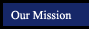Algebra I Structure and Method
Solving Equations and Problems Chapter 3
 Section Title Tutorials from this book More Examples (in pop-up window) 3.1 Transforming Equations: Addition and Subtraction 1 5 9 15 23 a b c d e Practice Game -Solving 1-Step Equations Using Addition and Subtraction f g Practice Game -Solving 1-Step Equations Using Addition and Subtraction (With Fractions!) Type 1 Practice Game -Solving 1-Step Equations Using Addition and Subtraction (With Fractions!) Type 2 3.2 Transforming Equations: Multiplication and Division 1 19 23 27 29 31 33 a b c d e Practice Game -Solving 1-Step Equations Using Multiplication and Division f g h Practice Game - Solving 1-Step Equations with Fractions Next to the Variable 1 Step Equation Practice Activiity (random mix of addition, subtraction, multiplication and division) 3.3 Using Several Transformations 1 7 9 11 13 17 23 27 31 35 a b c d e 2 Step Equation Practice Activity f g h i 3.4 Using Equations to Solve Problems 1 5 13 15 3.5 Equations with the Variable on Both Sides 1 9 15 21 27 a b c d word problems 1 5 9 15 Practice Game - Equations With Variables on Both Sides - Level 0 Practice Game - Equations With Variables on Both Sides - Level 1 Practice Game - Equations With Variables on Both Sides - Level 2 Practice Game - Variable on Both Sides, With Parentheses Practice Game - Variable and Parentheses on Both Sides 3.6 Problem Solving: Using Charts 1 5 7 3.7 Cost, Income, and Value Problems 1 3 5 3.8 Proof in Algebra